Vectors and conics

This free course is available to start right now. Review the full course description and key learning outcomes and create an account and enrol if you want a free statement of participation.

Free course

2.2 Multiplication by a scalar

In the collection of vectors sketched in Section 2.1, although v is not equal to c, the vectors v and c are closely related: c is a vector in the same direction as v, but it is twice as long as v. Thus it is natural to write 2v for c, since we can think of a journey represented by c as being a journey v followed by a second journey v.

In an analogous way, we can write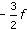for e, the vector whose magnitude istimes that of f and whose direction is that of −f.

Scalar multiple of a vector

Let k be a scalar and v a vector. Then kv is the vector whose magnitude is |k| times the magnitude of v, that is, ‖kv‖ = |k| ‖v‖, and whose direction is

the direction of v if k > 0,

the direction of −v if k < 0.

If k = 0, then kv = 0.

Example 19

For each of the vectors shown below, decide whether it is a multiple of any of the other vectors; if it is, write down an equation of the form v1 = kv2 that specifies the relationship between them.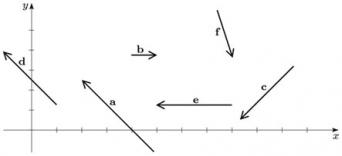The vector d is in the same direction as a, but none of the other vectors is; also, the length of d is two-thirds that of a. Hence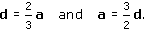Next, e is along the same line as b but in the opposite direction; none of the others is along the same line. Also, the length of e is three times that of b. Hence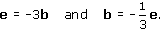Finally, c and f are not multiples of any of the other vectors.

Example 20

For the vector d in Exercise 19, sketch 3d and −2d.

The vector 3d is in the same direction as d, but its magnitude is three times that of d; the vector −2d is in the direction opposite to that of d, and its magnitude is twice that of d.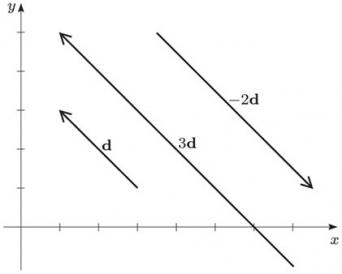M208_1